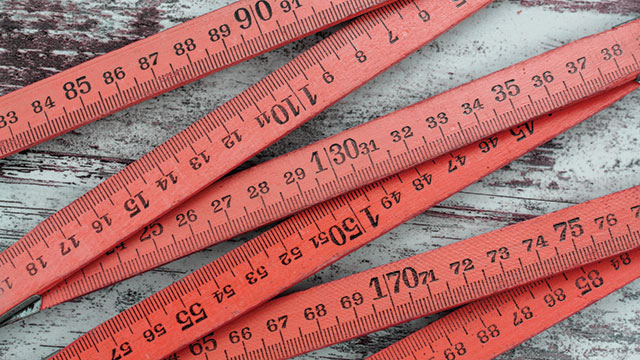top of page# General Conversion Table

There is more to measurements than just cups and teaspoons.

## Area

1 acre = 160 square rods = 43,560 square feet

1 square rod = 272.25 square feet = 16.5 feet x 16.5 feet

1 square foot = 144 square inches

1 foot = 12 inches

## Weight

1 ton = 2,000 pounds

1 pound = 16 ounces = 453.57 grams

1 ounce = 28.35 grams

1 gram = 15.43 grains

100 grams = 3.257 ounces

## Length

1 mile = 1,760 yards = 5,208 feet = 1,609.3 meters

1 yard = 3 feet = 36 inches = 0.9144 meters

1 foot = 12 inches = 30.480 centimeters

1 inch = 2.54 centimeters

1 meter = 39.37 inches = 100 centimeters

1 rod = 16.5 feet

## Liquid

1 gallon = 4 quarts = 128 fluid ounces = 231 cubic inches

1 quart = 2 pints = 32 fluid ounces = 57.75 cubic inches

1 pint = 2 cups = 16 fluid ounces = 32 tablespoons

1 cup = 237 milliliter = 8 fluid ounces = 16 tablespoons

1 ounce = 29.57 milliliter = 1 fluid ounce = 2 tablespoons

1 tablespoon = 15 milliliter = ½ fluid ounce = 3 teaspoons

1 cubic foot = 1,728 cubic inches

1 acre foot of water = 328,012 gallon = 2,722,500 pounds

1 acre foot of flow = 450 gallons per minute = 1 second foot for 12 hours

1 cubic foot/second (one second foot) = 450 gallons per minute

1 cubic foot = approximately 7.5 gallons

1 acre foot = 43,560 cubic foot.

Programs

Locations

Volunteers